MATLAB Function Referenceellipke

Complete elliptic integrals of the first and second kind

Syntax

• ```K = ellipke(M)
[K,E] = ellipke(M)
[K,E] = ellipke(M,tol)
```

Definition

The complete elliptic integral of the first kind  is

•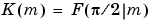where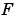, the elliptic integral of the first kind, is

•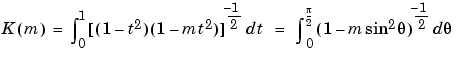The complete elliptic integral of the second kind

•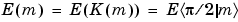is

•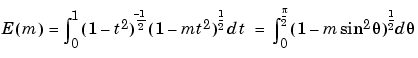Some definitions of `K` and `E` use the modulus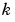instead of the parameter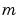. They are related by

•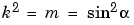Description

```K = ellipke(M) ``` returns the complete elliptic integral of the first kind for the elements of `M`.

```[K,E] = ellipke(M) ``` returns the complete elliptic integral of the first and second kinds.

```[K,E] = ellipke(M,tol) ``` computes the complete elliptic integral to accuracy `tol`. The default is `eps`; increase this for a less accurate but more quickly computed answer.

Algorithm

`ellipke` computes the complete elliptic integral using the method of the arithmetic-geometric mean described in , section 17.6. It starts with the triplet of numbers

•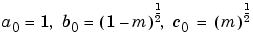`ellipke` computes successive iterations of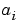,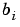, and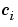with

•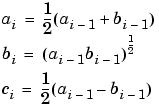stopping at iteration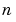when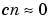, within the tolerance specified by `eps`. The complete elliptic integral of the first kind is then

•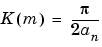Limitations

`ellipke` is limited to the input domain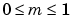.

See Also

`ellipj`

References

  Abramowitz, M. and I.A. Stegun, Handbook of Mathematical Functions, Dover Publications, 1965, 17.6.

© 1994-2005 The MathWorks, Inc.